• /*在Array和String类型之间转换，转换为字符串数组可以直接在URL上传递*/// convert a multidimensional array to url save and encoded string// usage: string Array2String( array Array )function Array2...

/*在Array和String类型之间转换，转换为字符串的数组可以直接在URL上传递*/

// convert a multidimensional array to url save and encoded string

// usage: string Array2String( array Array )

function Array2String($Array) {$Return='';

$NullValue="^^^"; foreach ($Array as $Key =>$Value) {

if(is_array($Value))$ReturnValue='^^array^'.Array2String($Value); else$ReturnValue=(strlen($Value)>0)?$Value:$NullValue;$Return.=urlencode(base64_encode($Key)) . '|' . urlencode(base64_encode($ReturnValue)).'||';

}

return urlencode(substr($Return,0,-2)); } // convert a string generated with Array2String() back to the original (multidimensional) array // usage: array String2Array ( string String) function String2Array($String)

{

$Return=array();$String=urldecode($String);$TempArray=explode('||',$String);$NullValue=urlencode(base64_encode("^^^"));

foreach ($TempArray as$TempValue) {

list($Key,$Value)=explode('|',$TempValue);$DecodedKey=base64_decode(urldecode($Key)); if($Value!=$NullValue) {$ReturnValue=base64_decode(urldecode($Value)); if(substr($ReturnValue,0,8)=='^^array^')

$ReturnValue=String2Array(substr($ReturnValue,8));

$Return[$DecodedKey]=$ReturnValue; } else$Return[$DecodedKey]=NULL; } return$Return;

}

?>

展开全文• 进入 PHP implode()的用法和explode()类似，但是功能却恰恰相反，implode()是将数组元素组合成一个字符串。 ?php $arr=array('a','b','c','d','e')；$s=implode($arr)； //默认是以空字符合并欢迎进入Linux社区论坛... 欢迎进入Linux社区论坛，与200万技术人员互动交流 >>进入 PHP implode()的用法和explode()类似，但是功能却恰恰相反，implode()是将数组元素组合成一个字符串。 ?php$arr=array('a','b','c','d','e')； $s=implode($arr)； //默认是以空字符合并

欢迎进入Linux社区论坛，与200万技术人员互动交流 >>进入

PHP implode()的用法和explode()类似，但是功能却恰恰相反，implode()是将数组元素组合成一个字符串。

$arr=array('a','b','c','d','e')；$s=implode($arr)； //默认是以空字符合并数组元素 //$s=implode(',',$arr)； //指定以","合并数组元素 echo$s;

?> 上面这是一个简单的一维数组，那么多维数组行不行呢？

$arr=array('a','b',array('4','5','6')，'c','d','e',array('1','2','3'))； //定义一个二维数组$s=implode(',',$arr)； echo$s;

?> 得到的结果是：

a,b,Array,c,d,e,Array 很显然，对于多维数组，implode()函数就无能为力了！那么多维数组怎样转字符串呢？如果先将多维数组转成一维数组，再通过implode()函数合并成字符串，不就可以完成了吗！多维数组转一维数组，在""一文中有详细的介绍，只要转成一维数组，其它的就简单了。展开全文• php将多维数组转字符串的方法：首先定义一个变量，一个数组变量；然后使用php的“json_encode”方法把二维数组转换为json字符串；最后使用“var_dump”方法输出转换后的结果即可。php把二维数组生成带有{}的字符串在...

php将多维数组转字符串的方法：首先定义一个变量，一个数组变量；然后使用php的“json_encode”方法把二维数组转换为json字符串；最后使用“var_dump”方法输出转换后的结果即可。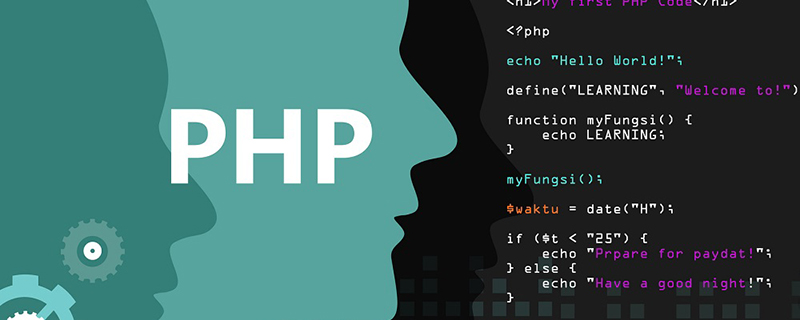php把二维数组生成带有{}的字符串

在php中，我们先定义一个变量，一个数组变量。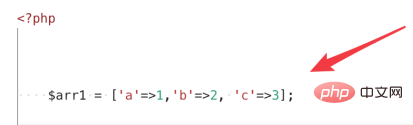另外再定义二个数组变量，现在有三个数组变量了。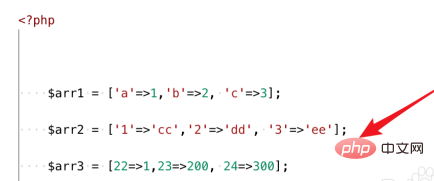接着再定义一个数据变量，但这个变量的数组值就是之前定义的那些数组，也就是说这个变量是一个二维数组。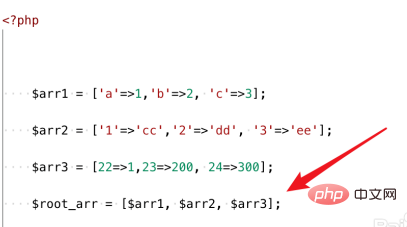要把二维数组转换为json字符串，可以使用php的json_encode方法。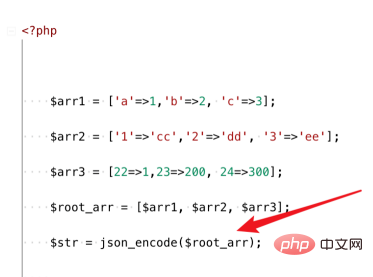最后我们使用var_dump方法输出转换后的结果。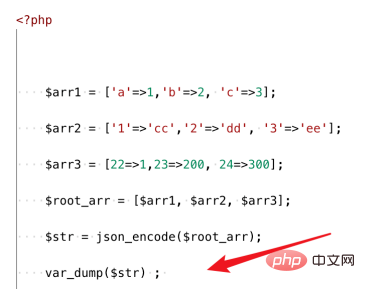运行页面，可以看到现在转换后的json字符串了，里面就是带有大括号{}的字符串。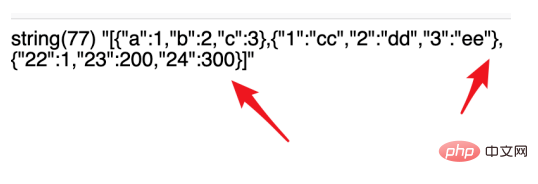要把这个json字符串转换回数组，我们可以使用php的json_decode方法。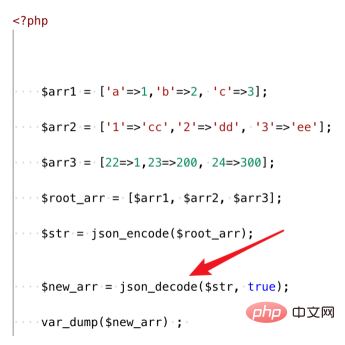转换后的结果就是一个二维数组。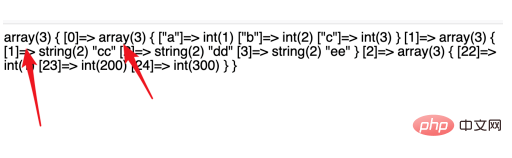展开全文• /*** @method 多维数组转字符串* @staticvar array $result_array* @param type$array* @return type* @link www.jquerycn.cn*/function arrayToString($arr){if (is_array($arr)){return implode(',', array_map('...

/**

* @method 多维数组转字符串

* @staticvar array $result_array * @param type$array

* @return type

*/

function arrayToString($arr) { if (is_array($arr))

{

return implode(',', array_map('arrayToString', $arr)); } return$arr;

}

/**

* @method 多维数组变成一维数组

* @staticvar array $result_array * @param type$array

* @return type

*/

function multi2array($array) { static$result_array = array();

foreach ($array as$key => $value) { if (is_array($value)) {

array_multi2array($value); } else$result_array[$key] =$value;

}

• JS数组转字符串（3种方法）

千次阅读 2021-01-08 14:17:10
JavaScript 允许数组字符串之间相互转换。其中 Array 方法对象定义了 3 个方法，可以把数组转换为字符串，如表所示。 Array 对象的数组字符串相互转换方法 数组方法 说明 toString() 将数组转换成一个字符串 ...
• List<OrderEntity> list = new List<OrderEntity>(){ new OrderEntity(){ Id=1001, Line=1 ,Item="TEST1"}, new OrderEntity(){ Id=1002, Line=2 ,Item="TEST2"}, new OrderEntity(){ Id=1003, Line=...c# 数组 字符串
• => string(4) "0.00" ["id"]=> string(1) "7" } => array(2) { ["money"]=> string(4) "0.00" ["id"]=> string(1) "4" } } } } } 例如将多维数组转成二维数组： $lis = array();$liss['money'] = \$list['money']...
• 这篇文章主要为大家详细介绍了php将一维或多维数组连接成一个字符串的简单示例，具有一定的参考价值，可以用来参考一下。感兴趣的小伙伴，下面一起跟随512笔记的小编小韵来看看吧！代码如下:/** ————————...
• 小编典典尝试我的stringToDeep()方法转换回Array。import java.util.*;public class DeepToArray {public static void main(String[] args) {int row, col;row = 2;col = 3;String[][] in = new String[row][col];...
• int main(int argc, const char * argv[]){@autoreleasepool {#pragma mark-----------二维数组int a={{1,2,3,4},{5,6,7,8},{12,10,11,12}};//打印二维数组for (int i = 0; i<3; i++) {for (int j =0; j<...
• 数组基础在C / C ++中，我们可以用简单的单词将多维数组定义为数组数组。多维数组中的数据以表格形式（以行主要顺序）存储。 声明N维数组的一般形式： data_type array_name [size1] [size2] .... [sizeN]; data_...数组 c语言 c++ 二维数组 多维数组
• 本篇文章将介绍JS数组转字符串的三种方法。 文章目录一、toString()方法二、toLocalString()方法三、join() 方法 JavaScript 允许数组字符串之间相互转换。因此提供了三种方法用来进行转换。 以下开始分别介绍...javascript 前端 开发语言
• //[1,2,3,4,5,6] 四、flat方法 数组的flat方法用于返回一个新数组，不改变原数组。 参数接受一个数字，默认为1，进行一次扁平化 const arr = [1,2,3,[[4,4,4],5],[6,7]]; const arr1 = arr.flat(); console.log(arr...javascript
• 我试图使用ctypes将字符数组数组传递给C函数。void cfunction(char ** strings){strings = "bad"; //works not what I need.strings = 'd'; //this will segfault.return;}char *input[] = {"foo","bar"};c...
• #-*- coding=utf-8 -*-listm=["s","l","z","x","l","m","XX",[123,23,4,89,05,[78,35,65,2017],"zX",1.2],2.3,4.5,6.7,8.9,120.23,34.45]int_num=0float_num=0letter_num=0for i in listm:if isinstance(i,list):for...
• 对象转换成字符串需要使用toString()方法。1 var a = function(){2 console.log(111);3 };4 var b = a.toString();5 console.log(a); 函数式的function6 console.log(b); 字符串function7 console.log(typeof a); &...
• reduce()方法对数组中的每个元素执行一个由您提供的reducer函数(升序执行)，将其结果汇总为单个返回值。语法为： array.reduce(function(accumulator, currentValue, currentIndex, arr), initialValue); /* ...vue
• C语言总结第七章、数组一维数组一维数组的定义一维数组的引用一维数组的初始化程序举例二维数组及多维数组二维数组的定义二维数组元素的引用二维数组元素的初始化程序举例字符数组和字符串字符数组字符串第七章、...
• 目标：学习并了解数组字符串的基本内容与含义，并且可以进行简单的程序设计和解决有关数组字符串的问题。 内容：1.给你一个整数数组 nums，请编写一个能够返回数组 “中心下标” 的方法。数组 中心下标 是数组的...字符串 数组
• 多维数组 字符数组和字符串 字符数组 字符串 二维字符串数组 数组简述 数组是构造类型之一 数组是具有一定顺序关系的若干个变量的集合，组成数组的各个变量称为数组的元素。 数组中个元素的数据类型要求相同...c语言 数组 字符串
• 实验报告 Java数组与字符串实验五 Java数组与字符串【实验目的】掌握数组的声明、分配空间及初始化理解多维数组(重点掌握二维)掌握String类的特点及常用方法的使用掌握StringBuffer类的特点及常用方法的使用掌握......